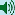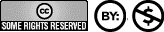### H2 - The Sound of Hydrogen Molecules

Hydrogen is the most common element in the universe. Thus, the universe is most permeated by the vibrational structures of hydrogen spectra. Hydrogen spectra are universal harmonic structures.

##### Spectrum of the Rainbow

In general, the stepless sequence of rainbow colors (red, orange, yellow, green, blue and violet) is called a continuous spectrum. In 1802, the English physician, chemist and physicist William Hyde Wollaston discovered dark lines on the continuous color background of the solar spectrum when he looked closely at the beam of sunlight split by a prism. In 1814, the physicist and astronomer Joseph Fraunhofer labeled these lines with Latin letters. Of the dozen or so most conspicuous lines in the solar spectrum, four were later clearly identified as hydrogen absorption lines. Therefore it is known that the sun is surrounded by hydrogen gas.

The significance of these dark lines in the solar spectrum did not become clear until 1859, when two physicists, Robert Wilhelm Bunsen, the inventor of the Bunsen burner, and Gustav Robert Kirchhoff, who were friends, established the fundamentals of spectral analysis in laboratory experiments.

Spectral analysis is a physical examination method in which the chemical composition of the light source or absorbing media in question can be deduced from the lines of an emission or absorption spectrum. From the position of the lines (characterized by their wavelengths) the element in question can be identified, from their intensity the quantity, from their splitting and distribution the atomic and molecular structure..

A wave is characterized by its wavelength, its intensity, the oscillation amplitude, or amplitude, and by its propagation speed. The propagation speed of light is 2.997 925-1010 cm per second and is called the speed of light. If we consider any point of a wave, it performs a time-dependent regular oscillation. The frequency of oscillation of such a point is called the frequency of the wave.

##### The Hydrogen Spectrum

The lines in the spectra of glowing gases often occur in mathematically easily describable series. The first series law was discovered for hydrogen, the smallest atom and first element of the periodic table of chemical elements, by the secondary school teacher Johann Jakob Balmer in Basel in 1885.

The wavelengths of the spectral lines of the hydrogen atom correspond to the following equation found by Balmer:

λ = R·[m2 / (m2 – 4)]

Here λ is the wavelength of the spectral line in nanometers (1 nm = 1 nanometer = 0.001 micrometer = 0.000 001 millimeter). R = 364.6 nm is a constant. The fundamental importance of this constant for the structure and determination of spectra was discovered by the Swedish physicist Johannes Robert Rydberg in 1889. The Rydberg constant R, named after him, corresponds to the measure of the so-called ionization energy that must be supplied to an electron in order to remove it from the atom in question. Let the value m in the formula be, in order, 3; 4; 5; 6 and 7 (a sequence of natural numbers). It is for:

m = 3 : R . (9/5)     = 656.470 nm    Hα (alpha)    orange
m = 4 : R . (4/3)     = 486.274 nm    Hβ (beta)      blue-green
m = 5 : R . (25/21) = 434.173 nm    Hγ (gamma ) blue-violet
m = 6 : R . (9/8)     = 410.294 nm    Hδ (delta)     violet
m = 7 : R . (49/45) = 397.124 nm    Hε (epsilon)   red-violet

The color circle-note-assignment and the color frequency-note-assignment are only approximately congruent. Therefore, other color names can be found in physical treatises: Hydrogen alpha: red instead of orange, hydrogen gamma: blue instead of blue-violet and hydrogen epsilon: ultraviolet instead of red-violet.

The harmonic structure of individual factors with which R is multiplied is striking here. 9/5 is the vibration ratio of the minor seventh, 4/3 that of the fourth and 9/8 that of the natural whole tone. It can be seen that also in the quantum leaps on the atomic level the proportions of the energy levels are oriented to classical harmonic principles.

For the conversion of the wavelengths into the corresponding frequencies, respectively of the frequencies into the corresponding wavelengths, the following two formulas are valid:

λ = c / f and f = c / λ

where λ is the wavelength, f is the frequency, and c is the speed of light [2.997 925·1017nm·sec-1]

Thus, the frequencies of the spectral lines of hydrogen have the following values:
Hα 456.674 ·1012 Hz
Hβ 616.510 ·1012 Hz
Hγ 690.491 ·1012 Hz
Hδ 730.678·1012 Hz
Hε 754.910·1012 Hz

The octavation (division by 241, respectively by 242) into the middle audible range leads to the following tone frequencies:Hα207.67 Hz (sample) = G#Hβ 140.18 Hz (sample) = C#Hγ 157.00 Hz (sample) = D#Hδ 166.14 Hz (sample) = EHε 171.65 Hz (sample) = F
(sound files each WAV 160 KB, 32.05 KHz sampling rate, 8 bit)

The first two lines of the hydrogen spectrum are octave analogous almost in accordance with the orbital periods of the planets Uranus and Pluto. The tone of Uranus sounds in the 39th octave at 207.36 Hz, so it is only a third of a hertz lower than the first hydrogen line, and the tone of Pluto sounds in the 40th octave at 140.25 Hz, so it is very precisely in line with the second spectral line of the hydrogen atom (note: more recent data from NASA on Pluto's solar orbital period give a Pluto tone of 140.65 Hz).

The visible spectrum of hydrogen is only a small part of the total hydrogen spectrum. Beyond the ultraviolet range lies the so-called Lymann series, beyond the infrared range lie the so-called Paschen, Backett and Pound series. These series contain partly exact octave analogous frequencies to the visible Balmer series.

Detailed information papers by Hans Cousto about the frequencies of all hydrogen series with tuning hints can be downloaded as PDF files (see above).

##### Detailed Documentations by Hans Cousto (in German)

All hydrogen series: Lyman, Balmer, Paschen, Brachett, Pfund

Part 1:
Musikalische Transkription der Wasserstoffspektren
Musical transcription of hydrogen spectra
Physikalischen Grundlagen zur Anhörung der Quantentheorie

Physical basics for hearing the quantum theory

Information booklet about all hydrogen frequencies and with voice technical hints.

Graphs of the difference tones of hydrogen spectral frequencies
(Section 3.7 from above hydrogen-1.pdf)

Part 2:
Stimmschlüssel für Akustiker
Tuning key for acoustician